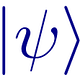# How to calculate the depth of a quantum circuit in Qiskit?

The depth of a circuit is a metric that calculates the longest path between the data input and the output. Each gate counts as a unit.

An important point to consider: if one qubit depends on another, one of them has to wait for the other to be computed. Whoever has the longest time will be the bottleneck.

Example 1:

In this case, the gates are in parallel. So, clearly the first qubit has two gates and the second, one.

In Qiskit, you can calculate the depth using the command:

`QuantumCircuit.depth`

Example 2:

In this case, the first qubit suffers the action of 4 Hadamards and then a CX: depth 5.

The second qubit, apparently, only suffers the action of CX and then H. However, as it has to wait for the first qubit (depth 5) before applying H, in fact, the depth is 6.

The following code, running on Qiskit, shows this.

`from qiskit import *`

`qc = QuantumCircuit(2)`

`qc.h(0)`

`qc.h(0)`

`qc.h(0)`

`qc.h(0)`

`qc.cx(0,1)`
`qc.h(1)`

`print(qc)`
`print("Circuit depth: ", qc.depth())`

The critical path is the computation of the 4 H’s in the first qubit, the CX, and then the H in the second qubit.

There are people who consider the measurement gate in the circuit depth, others do not. Qiskit considers the measurement.

Example 3: The best way to do the math is to consider each port at once. For each qubit affected by the gate, consider which has the longest path. It is the Dynamic Programming technique.

The circuit starts with depth
[0 0 0]

Applying each gate:

H 0: [1 0 0]
CX 0 1: [2 2 0] (Because CX affects 0 and 1, so you have to take the longest time between the two and add a unit)
H 0: [3 2 0]
H 1: [3 3 0]
CX 1 2: [3 4 4] (Because CX affects 1 and 2, so you have to take the longest time between the two and add a unit)

Therefore, the depth of the circuit is 4. For those who want to check this in qiskit, it is the following code.

`from qiskit import *`
`qc = QuantumCircuit(3)`

`qc.h(0)`
`qc.cx(0,1)`
`qc.h(0)`
`qc.h(1)`
`qc.cx(1,2)`

`print(qc)`
`print("Circuit depth: ", qc.depth())`

Note:

I’m creating a study group about Quantum Computing, to share news, events and to discuss ideias. It’s in Portuguese.

`https://quantumcomputing.stackexchange.com/questions/5769/how-to-calculate-circuit-depth-properly#:~:text=The%20circuit%20depth%20is%20the,in%20time%20along%20qubit%20wires.`

## Arnaldo Gunzi Quantum

Arnaldo Gunzi notes on Quantum Computing and Information

Written by

## Arnaldo Gunzi

Project Manager on Analytics and Innovation. “Samurai of Analytics”. Passionate about Combinatorial Optimization, Philosophy and Quantum Computing.## Arnaldo Gunzi Quantum

Arnaldo Gunzi notes on Quantum Computing and Information

## More From Medium

Medium is an open platform where 170 million readers come to find insightful and dynamic thinking. Here, expert and undiscovered voices alike dive into the heart of any topic and bring new ideas to the surface. Learn more

Follow the writers, publications, and topics that matter to you, and you’ll see them on your homepage and in your inbox. Explore

If you have a story to tell, knowledge to share, or a perspective to offer — welcome home. It’s easy and free to post your thinking on any topic. Write on Medium